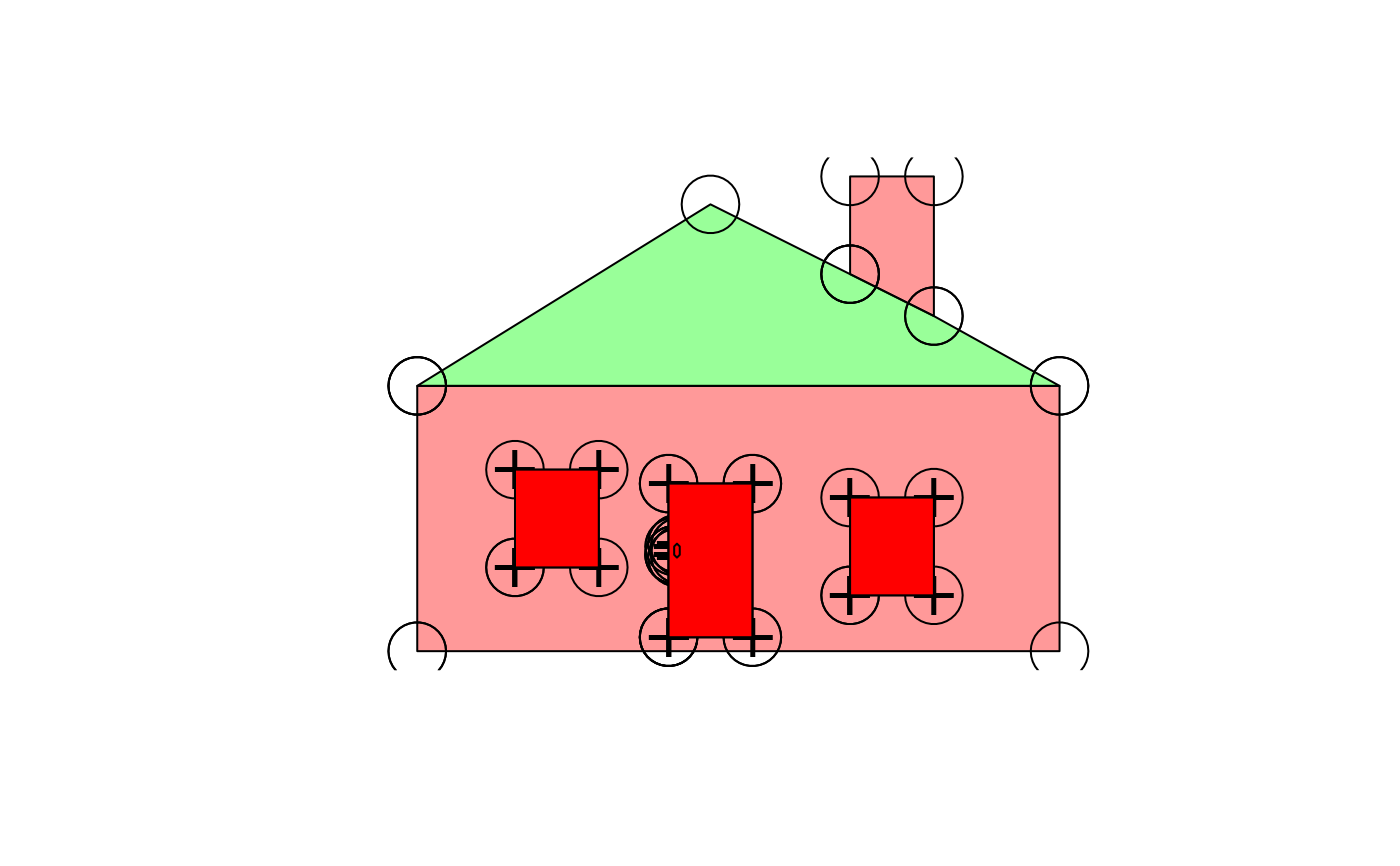Decompose a Spatial or sf object to a single table structured as a row for every coordinate in all the sub-geometries, including duplicated coordinates that close polygonal rings, close lines and shared vertices between objects.

# S3 method for SpatialPolygons
sptable(x, ...)

# S3 method for SpatialLines
sptable(x, ...)

# S3 method for SpatialPointsDataFrame
sptable(x, ...)

# S3 method for SpatialMultiPointsDataFrame
sptable(x, ...)

sptable(object) <- value

sptable(x, ...)

# S3 method for trip
map_table(x, ...)

## Arguments

x Spatial object ignored Spatial object modified sptable version of object

## Value

Spatial object

tibble with columns

• SpatialPolygonsDataFrame "object_" "branch_" "island_" "order_" "x" "y_"

• SpatialLinesDataFrame "object_" "branch_" "order_" "x_" "y_"

• SpatialPointsDataFrame "object_" x_" "y_"

• SpatialMultiPointsDataFrame "object_" "branch_" "x_" "y_"

• sf some combination of the above

## Details

Input can be a of type sf SpatialPolygonsDataFrame, SpatialLinesDataFrame, SpatialMultiPointsDataFrame or a SpatialPointsDataFrame. For simplicity sptable and its inverses sp and sf assume that all geometry can be encoded with object, branch, island, order, x and y. and that the type of topology is identified by which of these are present.

For simple features objects with mixed types of topology the result is consistent, but probably not useful. Columns that aren't present in one type will be present, padded with NA. (This is work in progress).

This is analogous to the following but in spbabel provides a consistent way to round-trip back to Spatial classes and other forms.

• sp_tidiers (replacement of 'ggplot2::fortify').

• geom

• SpatialPolygonsDataFrame-class with its 'as(as(x, "SpatialLinesDataFrame"), "SpatialPointsDataFrame")' work flow.

## Examples

## holey is a decomposed SpatialPolygonsDataFrame
spdata <- sp(holey)
library(sp)
plot(spdata, col = rainbow(nrow(spdata), alpha = 0.4))points(holey$x_, holey$y_, cex = 4)holes <- subset(holey, !island_)
## add the points that only belong to holes
points(holes$x_, holes$y_, pch = "+", cex = 2)
## manipulate based on topology
## convert to not-holes
notahole <- holes
notahole$island_ <- TRUE #also convert to singular objects - note that this now means we have an overlapping pair of polys #because the door had a hole filled by another object notahole$object_ <- notahole\$branch_
plot(sp(notahole), add = TRUE, col = "red")## example using in-place modification with sptable<-
library(maptools)#> Checking rgeos availability: FALSE
#>  	Note: when rgeos is not available, polygon geometry 	computations in maptools depend on gpclib,
#>  	which has a restricted licence. It is disabled by default;
#>  	to enable gpclib, type gpclibPermit()data(wrld_simpl)
spdata2 <- spdata
library(dplyr)
## modify the geometry on this object without separating the vertices from the objects
sptable(spdata2) <- sptable(spdata2) %>% dplyr::mutate(x_ = x_ + 10, y_ = y_ + 5)